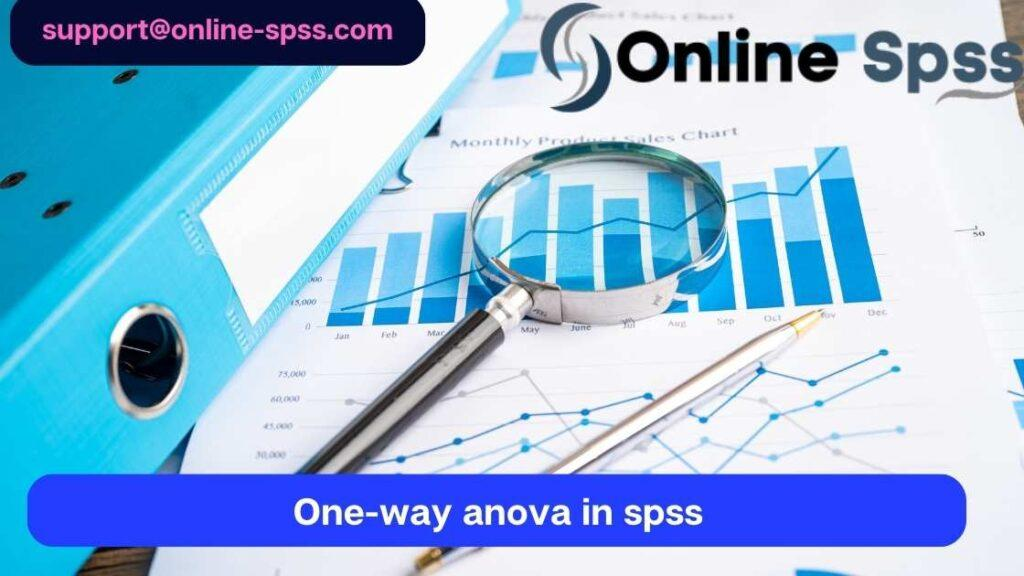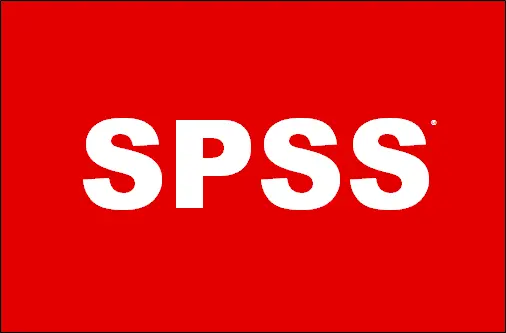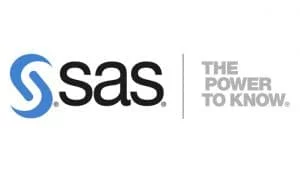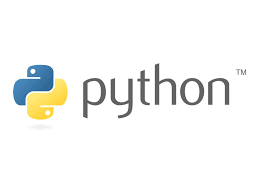# One Way Analysis of VarianceA One Way Analysis of Variance (ANOVA) is a statistical technique used to determine whether there exist a significant mean different between groups. It is mainly used when the number of independent groups (samples) are more than two. The specific criteria for using a one-way ANOVA is to check whether the number of independent groups being compared are more than 2 and whether there is one dependent variable measured on a  continuous scale.

This article provides a detailed discussion of a one-way ANOVA. It describe when to use a one-way ANOVA, when it is used, hypothesis testing in one-way ANOVA, the formula used to calculate the test statistic, as well as, the one-way ANOVA assumptions.

If you are familiar with the one-way ANOVA, but would like to learn how to perform ANOVA test in SPSS, go to our guide: Performing a One-way ANOVA in IBM SPSS.

## When to Use a One-Way ANOVA?

A one way analysis of variance (ANOVA) is used to determine whether there are any statistically significant differences between the means of three or more independent (unrelated) groups. It is widely used in various fields including science, psychology, biology, nursing, business, and economics, when there is a need to compared group means. A one-way ANOVA is an important statistical test because it help in comparing the means of three or more groups and determining whether there is a significant mean different between any two groups.

However, it is worth noting that a one-way ANOVA will only inform you whether there is a significant mean difference between any two groups. In other words, it does not indicates the specific groups with significantly different means. When it is established that there is a significant mean difference between any two groups, Post hoc analysis test comes in handy. Post hoc analysis test serves as a follow up test to identify the specific groups that significantly differ in the means.

## Hypothesis Testing in One Way Analysis of Variance

As highlighted above, a one-way ANOVA compares the means between3 or more unrelated groups. It aims to determines whether any of those group means are significantly different from each other. Specifically, the null and alternative hypotheses in one-way ANOVA are:

H0: The means in all the groups are equal (Not significantly different)

H1: At least two means are significantly different.

Therefore, if the one-way ANOVA reject the null hypothesis at a pre-defined significance level, we conclude that there is a significant mean difference between any two groups.

At this point, it is important to realize that the one-way ANOVA is an omnibus test statistic and cannot tell you which specific groups were statistically significantly different from each other, only that at least two groups were. To determine which specific groups differed from each other, you need to use a post hoc test.

### 15% OFF On Your 1st Order

Greetings! looking for professional data analysis services, SPSS Help or statistics homework help? Online-spss.com is your go-to destination for reliable data analysis help online. Get 15% off when you place an order.

## One-Way ANOVA Test Statistic Formula

To conduct a one way analysis of variance by hand, you need to calculate Within-groups sum of squares (SSW) and Between-groups sum of squares (SSB).

Next, you need to calculate the mean sum of squares within-groups (MSW) by dividing the within-groups sum of squares by the corresponding degrees of freedom (n-k).

Next, calculate the mean sum of squares between-groups (MSB) by dividing the between-groups sum of squares (SSB) by the corresponding degrees of freedom (k-1).

Where, n is the total sample size and k is the number of independent groups being compared.

Once, you have computed both MSW and MSB, then the test statistic is given by;

F = MSB/MSW

In this formula,

• F is the test statistic
• MSB = Mean sum of squares between the groups
• MSW = Mean sum of squares within groups

To make decision and conclusion about the one-way ANOVA hypothesis, you compared the computed test statistic (F) with the critical value (Fα, k-1, n-k).

If the test statistic (F) is greater than the critical value, (Fα, k-1, n-k), we reject the null hypothesis. Otherwise do not reject the null hypothesis.

If the null hypothesis is rejected, then we say that “there is sufficient evidence to conclude that there is a significant mean difference between any two groups” After making this decision, you need to perform post hoc test to determine which among the independent groups are statistically significantly different.

If you are familiar with running one-way ANOVA test in SPSS and need help on how to report the one-way ANOVA results, check out this article. Reporting One-Way ANOVA results in APA.

## Assumptions in One-Way ANOVA

In practice, checking for these six assumptions just adds a little bit more time to your analysis, requiring you to click a few more buttons in SPSS Statistics when performing your analysis, as well as think a little bit more about your data, but it is not a difficult task. Before we introduce you to these six assumptions, do not be surprised if, when analyzing your own data using SPSS Statistics, one or more of these assumptions is violated (i.e., is not met). This is not uncommon when working with real-world data rather than textbook examples, which often only show you how to carry out a one-way ANOVA when everything goes well! However, don’t worry. Even when your data fails certain assumptions, there is often a solution to overcome this. First, let’s take a look at these six assumptions: [continue with the rest of the text].

First, let’s take a look at these six assumptions:

1. Normality: The data should be normally distributed.
2. Independence: The observations should be independent of each other.
3. Homogeneity of variance: The variances of the groups being compared should be equal.
4. Linearity: There should be a linear relationship between the dependent and independent variables.
5. Absence of multicollinearity: The independent variables should not be highly correlated with each other.
6. Absence of outliers: There should be no extreme values that significantly affect the results.

Checking for these assumptions may require additional steps and analysis in SPSS Statistics. Violations of these assumptions are not uncommon, especially when working with real-world data. However, there are strategies to address the violations and still obtain meaningful results.

## GET HOMEWORK HELP FROM ONLINE-SPSS

There are various statistical software available today. Among these statistical software, SPSS is the most widely used, especially by university students when writing their thesis and dissertation papers. If you’re an Undergraduate student, a Master’s student, a PhD student, you must have encountered data analysis using SPSS and if not yet, then you will before the end of your studies.

Despite its significance in most fields, running data analysis using SPSS is often challenging. This is where Online-SPSS comes in. If you’re a student in need of  help with SPSS, then we got you covered. Our professional team of data analysis experts work tirelessly to help you offer you with SPSS data analysis assistance you may need.

Whether you are looking for spss help for dissertation, or spss homework help, our SPSS tutors will provide you with step-by-step solutions that guarantee an A+ grade. We understand that performing statistical analysis using SPSS by yourself is always cheaper. However, it can also be time-consuming, which can even lead to poor grades.

If you’re not proficient in SPSS, then performing statistical analysis using SPSS is not a good idea. It can take days just to figure out how to do some of the easier things in SPSS. So paying someone to do your SPSS will save you a huge amount of time and make your life a lot easier.

The procedure of getting SPSS help service at Online-SPSS.com is fairly simple. You only need to follow the following simple steps;

1. Click the ORDER NOW button
2. Make Secure Payment
3. Get the Solution

Our main focus is to provide quick, reliable, and professional data analysis services using SPSS and other statistical software.

### 15% OFF On Your 1st Order

Greetings! looking for professional data analysis services, SPSS Help or statistics homework help? Online-spss.com is your go-to destination for reliable data analysis help online. Get 15% off when you place an order.

### We Are Expert In:

With online-spss, you get statistical and data analysis help services from expert statisticians. We can help you with statistical analysis using the following statistical tools: